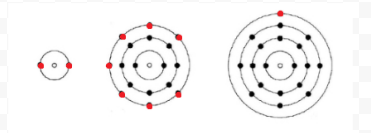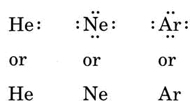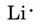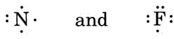# 5.3: Lewis Diagrams

$$\newcommand{\vecs}{\overset { \rightharpoonup} {\mathbf{#1}} }$$ $$\newcommand{\vecd}{\overset{-\!-\!\rightharpoonup}{\vphantom{a}\smash {#1}}}$$$$\newcommand{\id}{\mathrm{id}}$$ $$\newcommand{\Span}{\mathrm{span}}$$ $$\newcommand{\kernel}{\mathrm{null}\,}$$ $$\newcommand{\range}{\mathrm{range}\,}$$ $$\newcommand{\RealPart}{\mathrm{Re}}$$ $$\newcommand{\ImaginaryPart}{\mathrm{Im}}$$ $$\newcommand{\Argument}{\mathrm{Arg}}$$ $$\newcommand{\norm}{\| #1 \|}$$ $$\newcommand{\inner}{\langle #1, #2 \rangle}$$ $$\newcommand{\Span}{\mathrm{span}}$$ $$\newcommand{\id}{\mathrm{id}}$$ $$\newcommand{\Span}{\mathrm{span}}$$ $$\newcommand{\kernel}{\mathrm{null}\,}$$ $$\newcommand{\range}{\mathrm{range}\,}$$ $$\newcommand{\RealPart}{\mathrm{Re}}$$ $$\newcommand{\ImaginaryPart}{\mathrm{Im}}$$ $$\newcommand{\Argument}{\mathrm{Arg}}$$ $$\newcommand{\norm}{\| #1 \|}$$ $$\newcommand{\inner}{\langle #1, #2 \rangle}$$ $$\newcommand{\Span}{\mathrm{span}}$$$$\newcommand{\AA}{\unicode[.8,0]{x212B}}$$

Lewis used simple diagrams (now called Lewis diagrams) to keep track of how many electrons were present in the outermost, or valence, shell of a given atom. The kernel of the atom, i.e., the nucleus together with the inner electrons, is represented by the chemical symbol, and only the valence electrons are drawn as dots surrounding the chemical symbol. Thus the three atoms shown in Figure 1 from Electrons and Valence can be represented by the following Lewis diagrams:Atomic shells of helium, chlorine, and potassium showing all shells and the corresponding number of electrons.Figure $$\PageIndex{1}$$ The figure above shows the electron shells of He (Helium), Cl (Chlorine), and K (Potassium) as well as their Lewis dot structures below. Notice how both the electron shell and the lewis dot structures have the same number of valence electrons. The lewis dot structure ignores the nucleus and all non-valence electrons, displaying only the valence electrons of an atom.

If the atom is a noble-gas atom, two alternative procedures are possible. Either we can consider the atom to have zero valence electrons or we can regard the outermost filled shell as the valence shell. The first three noble gases can thus be written as:Helium is shown as H e alone or H e with two dots on the right. Neon is shown as N e alone or N e with two dots on the left, top, right, and bottom respectively. Argon is shown as A r alone or A r with two dots on the left, top, right, and bottom respectively.
##### Example $$\PageIndex{1}$$: Lewis Structures

Draw Lewis diagrams for an atom of each of the following elements: Li, N, F, Na

###### Solution

We find from the periodic table inside the front cover that Li has an atomic number of 3. It thus contains three electrons, one more than the noble gas He. This means that the outermost, or valence, shell contains only one electron, and the Lewis diagram isFollowing the same reasoning, N has seven electrons, five more than He, while F has nine electrons, seven more than He, givingNitrogen is N with two dots on the left and one dot on the top, right, and bottom respectively. Fluorine is F with two dots on the left, top, and right, and 1 dot below.

Na has nine more electrons than He, but eight of them are in the kernel, corresponding to the eight electrons in the outermost shell of Ne. Since Na has only 1 more electron than Ne, its Lewis diagram isNotice from the preceding example that the Lewis diagrams of the alkali metals are identical except for their chemical symbols. This agrees nicely with the very similar chemical behavior of the alkali metals. Similarly, Lewis diagrams for all elements in other groups, such as the alkaline earths or halogens, look the same.Figure $$\PageIndex{1}$$ The image above demonstrates that for elements in the same group (like the alkali earth metals shown above), the lewis dot structure will be the same, except of course for the different element name. In the image above you see that every alkali earth metal has 2 valence electrons, each represented by a dot in the lewis dot structure.

The Lewis diagrams may also be used to predict the valences of the elements. Lewis suggested that the number of valences of an atom was equal to the number of electrons in its valence shell or to the number of electrons which would have to be added to the valence shell to achieve the electronic shell structure of the next noble gas. As an example of this idea, consider the elements Be and O. Their Lewis diagrams and those of the noble gases He and Ne areThe Lewis structures are H e, B e with two dots on its right, O with two dots on the left, top and bottom respectively. N e with two dots on the left, top, right and bottom respectively.

Comparing Be with He, we see that the former has two more electrons and therefore should have a valence of 2. The element O might be expected to have a valence of 6 or a valence of 2 since it has six valence electrons—two less than Ne. Using rules of valence developed in this way, Lewis was able to account for the regular increase and decrease in the subscripts of the compounds in the table found in the Valence section, and reproduced here. In addition he was able to account for more than 50 percent of the formulas in the table. (Those that agree with his ideas are shaded in color in the table. You may wish to refer to that table now and verify that some of the indicated formulas follow Lewis’ rules.) Lewis’ success in this connection gave a clear indication that electrons were the most important factor in holding atoms together when molecules formed.

Despite these successes, there are also difficulties to be found in Lewis’ theories, in particular for elements beyond calcium in the periodic table. The element Br (Z = 35), for example, has 17 more electrons than the noble-gas Ar (Z = 18). This leads us to conclude that Br has 17 valence electrons, which makes it awkward to explain why Br resembles Cl and F so closely even though these two atoms have only seven valence electrons.

Table $$\PageIndex{1}$$ Common Compounds
Element Atomic Weight Hydrogen Compounds Oxygen Compounds Chlorine Compounds
Hydrogen 1.01 H2 H2O, H2O2 HCl
Helium 4.00 None formed None formed None formed
Lithium 6.94 LiH Li2O, Li2O2 LiCl
Beryllium 9.01 BeH2 BeO BeCl2
Boron 10.81 B2H6 B2O3 BCl3
Carbon 12.01 CH4, C2H6, C3H8 CO2, CO, C2O3 CCl4, C2Cl6
Nitrogen 14.01 NH3, N2H4, HN3 N2O, NO, NO2, N2O5 NCl3
Oxygen 16.00 H2O, H2O2 O2, O3 <Cl2O, ClO2, Cl2O7
Fluorine 19.00 HF OF2, O2F2 ClF, ClF3, ClF5
Neon 20.18 None formed None formed None formed
Sodium 22.99 NaH Na2O, Na2O2 NaCl
Magnesium 24.31 MgH2 MgO MgCl2
Aluminum 26.98 AlH3 Al2O3 AlCl3
Silicon 28.09 SiH4, Si2H6 SiO2 SiCl4, Si2Cl6
Phosphorus 30.97 PH3, P2H4 P4O10, P4O6 PCl3, PCl5, P2Cl4
Sulfur 32.06 H2S, H2S2 SO2, SO3 S2Cl2, SCl2, SCl4
Chlorine 35.45 HCl Cl2O, ClO2, Cl2O7 Cl2
Potassium 39.10 KH K2, K2O2, KO2 KCl
Argon 39.95 None formed None formed None formed
Calcium 40.08 CaH2 CaO, CaO2 CaCl2
Scandium 44.96 Relatively Unstable Sc2O3 ScCl3
Titanium 47.90 TiH2 TiO2, Ti2O3, TiO TiCl4, TiCl3, TiCl2
Vanadium 50.94 VH2 V2O5, V2O3, VO2, VO VCl4, VCl3, VCl2
Chromium 52.00 CrH2 Cr2O3, CrO2, CrO3 CrCl3, CrCl2

This page titled 5.3: Lewis Diagrams is shared under a CC BY-NC-SA 4.0 license and was authored, remixed, and/or curated by Ed Vitz, John W. Moore, Justin Shorb, Xavier Prat-Resina, Tim Wendorff, & Adam Hahn.